# Geometry

To understand more about triangular geometry, we have to learn a few more terms.

## Midpoint

The Midpoint of a segment is the point that divides the segment into two congruent (identical) segments.

The mixpoint is:

$$(x_m,y_m) = (\cfrac{x_2+x_1}{2}, \cfrac{y_2+y_1}{2})$$## Median

A Median is a line segment that extends from the vertex (corner) of one side of the triangle to the midpoint of the other side.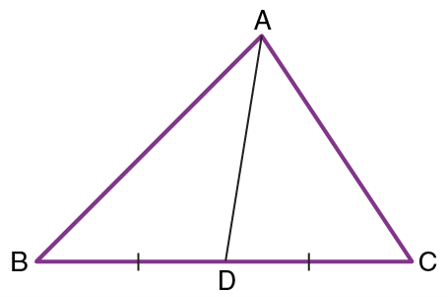## Midsegment

A Midsegment is a line segment connecting the midpoints of two sides of a triangle. it is also parallel to the third side of the triangle and is half of the length of the third side!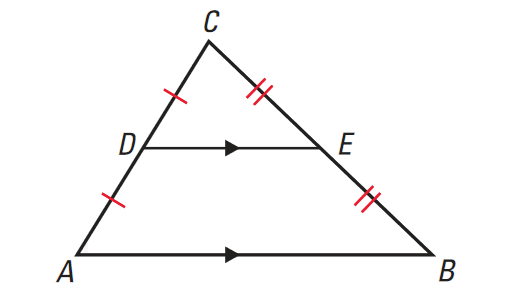## Altitude

An Altitude is a line segment that extends from the vertex (corner) of one side of the triangle and is perpendicular to the opposite line segment.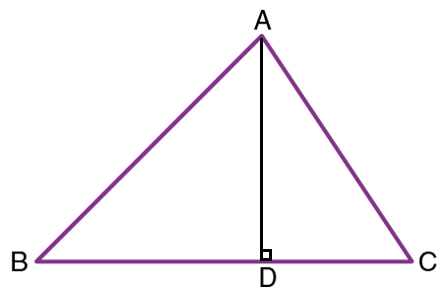## Perpendicular Bisector

The Perpendicular Bisector is a line or a segment perpendicular to a segment that passes through the midpoint of the segment. Then, the perpendicular bisector of a side of a triangle would be a line perpendicular to the side and passing through its midpoint. Since there are three perpendicular bisectors on the sides of a triangle, and they meet in a single point, that point would be called the circumcenter. The three perpendicular bisector of the triangle would converge at the center of the red circle.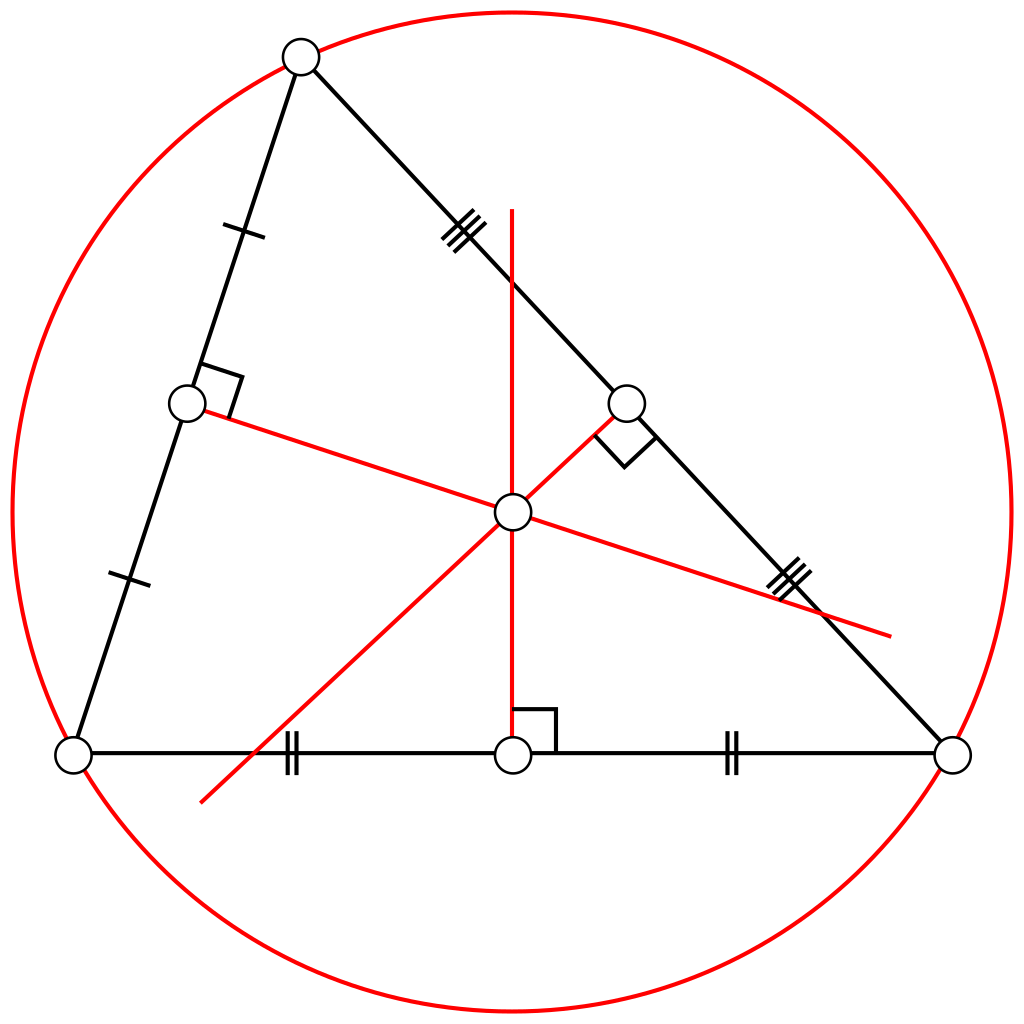## Angle Bisector

The Angle bisector of an angle of a triangle would be a straight line that divides the angle into two congruent (equal) angles. Since there are three angle bisectors on the sides of a triangle, and they meet in a single point, that point would be called the incenter.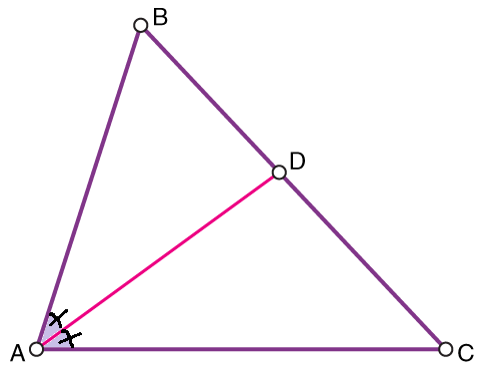A line is drawn from a vertext of a triangle to the midpoint of the opposite side. The line is a _____.

What is the difference between a median and altitude?

Given two points (1,5) and (5,-5). What's the midpoint between these two point?